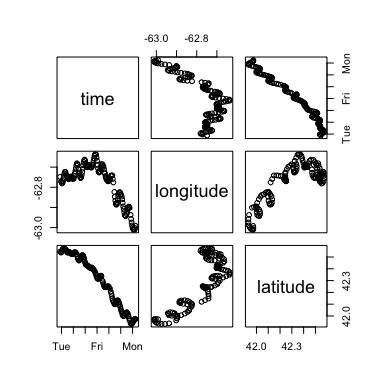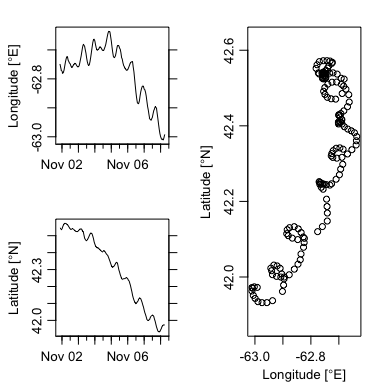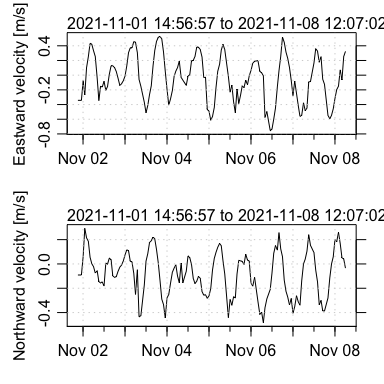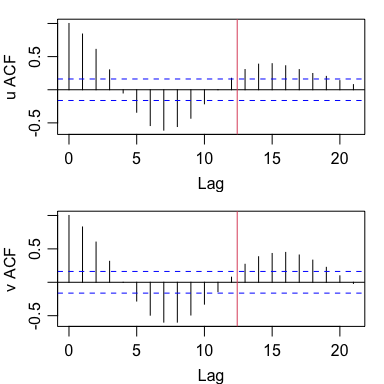# 6. Subclassing oce objects

#### 2022-08-18

Abstract. This vignette explains how to create new object classes, using the low-level oce class as a foundation. This scheme is beneficial, because the newly-formed objects automatically gain the key properties of oce objects, in terms of operators such as [[ and [[<-, functions such as subset() and summary(), schemes for handling units, etc. The setMethod() function is central to the process of creating new classes, as will be illustrated here in a practical way, using drifting-buoy data for example.

# 1 A drifting buoy

Data from buoy number 4201703 were downloaded from http://www.coriolis.eu.org/Data-Products/Data-selection on 2022-01-09, by deselecting all data types except for “Drifting buoy”, selecting a data window south of Nova Scotia and of area similar to that province, expanding the tarball created by the website and then focussing on the downloaded file named "drifting-buoys-4201703.csv“.

There are many data in the source data file, but only a subset is used here: starting with 300 samples of just five of the data fields: time (called t for brevity), longitude (lon), latitude (lat), temperature (Tatm) and atmospheric pressure (Patm). This set was further trimmed by removing data ensembles in which temperature was not reported. The result was 146 observations of 5 variables. (Note that they are not spaced uniformly in time, a feature that is of importance only at the end of this document.)

The data were combined into a data frame named db and packaged in an RDA file, an overview of which is provided as follows.

library(oce)
str(db)
#> 'data.frame':    146 obs. of  5 variables:
#>  $t : POSIXct, format: "2021-11-01 21:04:00" "2021-11-01 23:04:00" ... #>$ lon : num  -62.7 -62.8 -62.8 -62.8 -62.8 ...
#>  $lat : num 42.5 42.5 42.5 42.6 42.6 ... #>$ Tatm: num  19.2 19.2 19.2 19.2 19.2 ...
#>  $Patm: num 1013 1014 1015 1016 1017 ... Before showing how to create a new subclass, it will first be shown that such data could also be stored in the foundational class of the package. # 2 Using the base oce class The base class is named "oce", and so a new object of that type can be created with o <- new("oce") At this point, the summary() method does not reveal much: summary(o) #> * Processing Log #> #> - 2022-08-18 16:43:57 UTC: Create oce object but if we populate the object with some data and metadata o <- oceSetData(o, "time", db$t)
o <- oceSetData(o, "longitude", db$lon) o <- oceSetData(o, "latitude", db$lat)
o <- oceSetMetadata(o, "ID", 4201703)

summary(o)
#> * Time ranges from 2021-11-01 21:04:00 to 2021-11-08 06:00:00 with 146 samples and mean increment 1.054713 hour
#> * Data Overview
#>
#>                  Min.    Mean    Max. Dim. NAs OriginalName
#>     longitude -63.011 -62.775 -62.636  146   0            -
#>     latitude   41.932  42.305  42.572  146   0            -
#>
#> * Processing Log
#>
#>     - 2022-08-18 16:43:57 UTC: Create oce object
#>     - 2022-08-18 16:43:58 UTC: oceSetData(object = o, name = "time", value = db$t) #> - 2022-08-18 16:43:58 UTC: oceSetData(object = o, name = "longitude", value = db$lon)
#>     - 2022-08-18 16:43:58 UTC: oceSetData(object = o, name = "latitude", value = db$lat) #> - 2022-08-18 16:43:58 UTC: oceSetMetadata(object = o, name = "ID", value = 4201703) Note, however, that the ID is not listed by summary(). This is because the general form of that function is set up to focus on the contents of the data slot, not the metadata slot. (Extending summary() will described later.) Objects of oce-class automatically have other methods, besides summary(). For example, the accessing operator [[ works for the object that was just created: str(o[["latitude"]]) #> num [1:146] 42.5 42.5 42.5 42.6 42.6 ... We can also use the default plot() function to show the interdependence of entries in the data slot. plot(o)Figure 1. Demonstration of base-level plot(). Although this plot provides a useful overview of the data, it is not something an analyst would consider publishing. To get more useful plot types, we must create a new subclass that has a specialized plot() method. # 3 Creating a new subclass A reasonable name for our new subclass might be "drifter". With this choice, the class can be defined in a single line of code: drifter <- setClass(Class="drifter", contains="oce") Once this has been executed, the user can create a new object of this subclass with d <- new("drifter") Although it is simple to add data and metadata as in the previous section, it is more convenient to supply this information when new() is used. To accomplish this, we must set up an initialization function, as follows. setMethod(f="initialize", signature="drifter", definition=function(.Object, time, longitude, latitude, ID="unknown") { if (missing(time)) stop("In new(drifter) : must provide 'time'", call.=FALSE) if (missing(longitude)) stop("In new(drifter) : must provide 'longitude'", call.=FALSE) if (missing(latitude)) stop("In new(drifter) : must provide 'latitude'", call.=FALSE) .Object@data$time <- time
.Object@data$longitude <- longitude .Object@data$latitude <- latitude
.Object@metadata$ID <- ID .Object@processingLog$time <- presentTime()
.Object@processingLog$value <- "create 'drifter' object" return(.Object) }) This method takes away the choice of where to put things (in data or in metadata), saving users from having to make that decision, possibly breaking other code. Also, note that some error checking has been used here, so that an attempt to use new("drifter") without providing time, etc., produces an error message, as shown below. d <- new("drifter") Error: In new(drifter) : must provide 'time' With this setup, we may now create an object that we can use for the rest of this vignette: d <- new("drifter", time=db$t, longitude=db$lon, latitude=db$lat, ID=4201703)

and this will be used in the next three subsections, showing how to specialize the plot() method, the summary() method, and the [[ accessor. Note the use of setMethod() in each instance.

## 3.1 Specializing the plot() function

The f, signature and definition arguments follow the same pattern as in the previous example, and readers ought to focus on last of these. Here, three plot types are provided, as selected by an argument called which. The first two give time-series plots of drifter longitude and latitude, and the third shows the trajectory.

setMethod(f="plot",
signature=signature("drifter"),
definition=function(x, which=1, ...) {
lonlab <- expression("Longitude ["*degree*"E]")
latlab <- expression("Latitude ["*degree*"N]")
if (which == 1) {
oce.plot.ts(x[["time"]], x[["longitude"]],
ylab=lonlab, ...)
} else if (which == 2) {
oce.plot.ts(x[["time"]], x[["latitude"]],
ylab=latlab,...)
} else if (which == 3) {
asp <- 1 / cos(mean(range(x[["latitude"]]) * pi / 180))
plot(x[["longitude"]], x[["latitude"]],
asp=asp, xlab=lonlab, ylab=latlab, ...)
} else {
stop("In plot,drifter-method : try which=1, 2 or 3", call.=FALSE)
}
})

The three plot types are fairly rudimentary, but the ... argument can be used for customization, as in the following example.

par(mar=c(3.3,3.3,1,1), mgp=c(2,0.7,0))
layout(matrix(c(1,3,2,3),nrow=2,byrow=TRUE))
plot(d, which=1, drawTimeRange=FALSE)
plot(d, which=2, drawTimeRange=FALSE)
plot(d, which=3)Figure 2. Demonstration of specialized plot().

## 3.2 Specializing the summary() function

Here, our sole purpose is to add an indication of the ID of the drifter. The rest of the information provided by the base-level summary() function will suffice to summarize the rest.

So, with

setMethod(f="summary",
signature="drifter",
definition=function(object, ...) {
cat("CTD Summary\n-----------\n\n")
cat("* ID:          ", object[["ID"]], "\n", sep="")
invisible(callNextMethod())
})

An example follows.

summary(d)
#> CTD Summary
#> -----------
#>
#> * ID:          4201703
#> * Time ranges from 2021-11-01 21:04:00 to 2021-11-08 06:00:00 with 146 samples and mean increment 1.054713 hour
#> * Data Overview
#>
#>                  Min.    Mean    Max. Dim. NAs OriginalName
#>     longitude -63.011 -62.775 -62.636  146   0            -
#>     latitude   41.932  42.305  42.572  146   0            -
#>
#> * Processing Log
#>
#>     - 2022-08-18 16:43:58 UTC: create 'drifter' object

## 3.3 Specializing the [[ accessor

The variation of drifter position provides information on ocean velocity, and this is of sufficient interest that it might be worth adding to [[. This may be done as follows.

setMethod(f="[[",
signature(x="drifter", i="ANY", j="ANY"),
definition=function(x, i, j, ...) {
if (i == "velocity") {
D <- function(x) {
dx <- diff(x)
c(dx, dx)
}
dist <- geodDist(x[["longitude"]], x[["latitude"]])
lat <- x[["latitude"]]
lon <- x[["longitude"]]
dt <- D(as.numeric(x[["time"]])) # seconds
scalex <- 111.12e3         # m per degree latitude
scaley <- scalex * cos(lat*pi/180)
u <- scalex * D(lon) / dt
v <- scaley * D(lat) / dt
list(u=u, v=v)
} else {
callNextMethod()
}
})

As a test, we can plot the velocity components.

uv <- d[["velocity"]]
par(mfrow=c(2,1))
oce.plot.ts(d[["time"]], uv$u, ylab="Eastward velocity [m/s]", grid=TRUE) oce.plot.ts(d[["time"]], uv$v, ylab="Northward velocity [m/s]", grid=TRUE)Figure 3. Velocities inferred from drifter motion.

The acf() function, which computes lagged autocorrelation, can shed some light on the periodicity of the velocity signals (but see Exercise 3), as follows.

data(tidedata)
M2period <- 1 / with(tidedata$const, freq[[which(name=="M2")]]) uv <- d[["velocity"]] par(mfrow=c(2,1), mar=c(3,3,1,1), mgp=c(2,0.7,0)) acf(uv$u, main="", ylab="u ACF")
abline(v=M2period, col=2)
acf(uv\$v, main="", ylab="v ACF")
abline(v=M2period, col=2)Figure 4. Autocorrelation analysis of drifter velocities, showing also the M2 period.

# 4 Summary

As has been illustrated above, the setMethod() function is the key to altering generic functions such as plot(), summary() and [[. The details of how this works are explained in numerous manuals, books, and websites, but impatient readers can save some time by simply copying and expanding on the code provided in the previous sections.

# 5 Appendix: exercises for the reader

1. Add atmospheric temperature and pressure (both contained in the source RDA file) to the drifter object initialization function. Be sure to check that they were provided or, if you choose, let them be optional.

2. Think of other useful plot types, and add these to the plot() method.

3. For the final lagged-autocorrelation function, use approx() to get new time-series with a constant sampling rate.

4. Again for the lagged-autocorrelation function, add a line for local Coriolis period.

5. As an alternative to the lagged-autocorrelation method, test for periodicity of velocity by using nls(), supplied with a sinusoidal function that has adjustable amplitude, phase, and frequency.